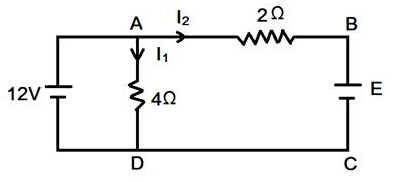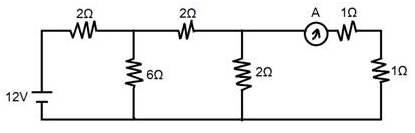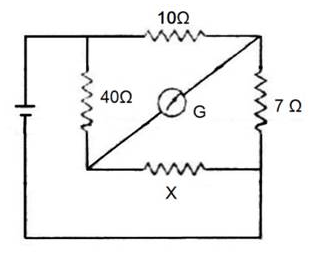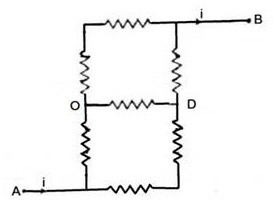# ELECTRIC CIRCUITS

• Home -
• ELECTRIC CIRCUITS

ELECTRIC CIRCUITS - Topic Wise Multiple Choice Questions (MCQ)

Topic Wise free Electric Circuits MCQ with solutions for 11th 12th exam Practice Multiple Choice Questions and answer for JEE-mains State CET Engineering Entrance GATE free Online Test

1 )

Two wires of equal lengths, equal diameters and having resistivities ρ1 and ρ2 are connected in series The equivalent resistivity of the combination is....

(ρ1 + ρ2)
(1/2) (ρ1 + ρ2)
{(ρ1ρ2) / (ρ1 + ρ2)}
√(ρ1ρ2)

View Solution

2 )

In the circuit shown in fig, current I2 = 0. The value of E is....3V
6V
9V
12V

View Solution

3 )

In the circuit shown in fig, the reading of ammeter is....1A
2A
3A
4A

View Solution

4 )

In Fig, the galvanometer shows no deflection. What is the resistance X?14Ω
21Ω
28Ω

View Solution

5 )

Seven resistors, each of resistance 5Ω, are connected as shown in fig, The equivalent resistance between points A and B is....35Ω
49Ω

View Solution